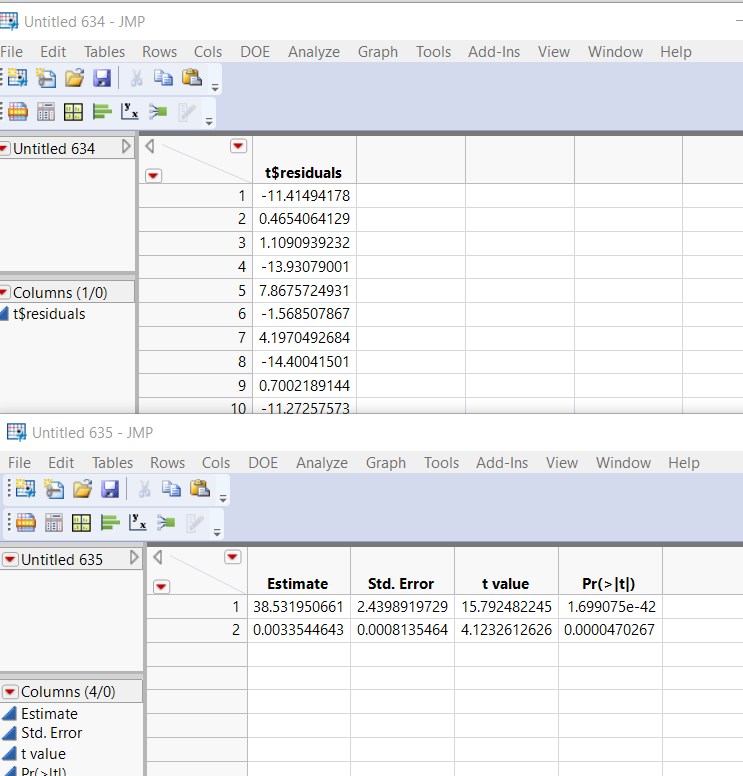Choose Language Hide Translation Bar
Highlighted

## How to get R standard output

When I submit R Code via JSL, the R console standard output (stdout) displays in the JMP log.  Is there a way to capture that information in a text string?  I know I can redirect the stdout from R to a file (using the sink() function) and then get the file contents in a JMP variable with some JSL, but I'd rather not have to use an intermediate step like that.

Example;

``````names default to here(1);

R Init();

dt=open("\$SAMPLE_DATA/Cars.jmp");
R Send(dt);

R Submit(
"
summary(lm(formula=Chest.decel ~ Wt, data=dt))
"
);

RTerm();``````

This output shows in the log file

``````Call:
lm(formula = Chest.decel ~ Wt, data = dt)

Residuals:
Min      1Q  Median      3Q     Max
-17.931  -6.650  -1.482   5.593  44.232

Coefficients:
Estimate Std. Error t value Pr(>|t|)
(Intercept) 3.853e+01  2.440e+00  15.792  < 2e-16 ***
Wt          3.354e-03  8.135e-04   4.123  4.7e-05 ***
---
Signif. codes:  0 '***' 0.001 '**' 0.01 '*' 0.05 '.' 0.1 ' ' 1

Residual standard error: 9.367 on 339 degrees of freedom
(11 observations deleted due to missingness)
Multiple R-squared:  0.04776,	Adjusted R-squared:  0.04495
F-statistic:    17 on 1 and 339 DF,  p-value: 4.703e-05``````

I want to be able to get the output as a text string and put that into a Text Box in a report window.

1 ACCEPTED SOLUTION

Accepted Solutions
Highlighted

## Re: How to get R standard output

Log Capture() may do what you want.

``````Names Default To Here(1);

R Init();

dt = Open("\$SAMPLE_DATA/Cars.jmp");
R Send(dt);

R_stdout = Log Capture(R Submit("
summary(lm(formula=Chest.decel ~ Wt, data=dt))
"));

R Term();

New Window("example", Text Box(R_stdout));``````
5 REPLIES 5
Highlighted

## Re: How to get R standard output

@MathStatChem ,
Before I go too far ahead, are you not wanting to use R Get() ? Did you try using R Get() and then using that information as input to the text box ?

Best
Uday
Highlighted

## Re: How to get R standard output

From what I can see, R Get() will get the values from an R data structure or variable (number, string, matrix, list, or data frame), but not the standard output.

Highlighted

## Re: How to get R standard output

Try this:

``````R Init();

dt=open("\$SAMPLE_DATA/Cars.jmp");
R Send(dt);

R Submit(
"
model = lm(Chest.decel ~ Wt,data = dt)
t = summary(model)

# Use names function to determine what are the objects that exist
names(t)

# Extract and cast into data frames
Residuals = as.data.frame(t\$residuals)
Coefficients = as.data.frame(t\$coefficients)

"
);

ResidJMP = R Get(Residuals);
ResidJMP << New Data View;
CoeffJMP = R Get(Coefficients);
CoeffJMP << New Data View;

RTerm();

Close(dt,"No Save");``````Best
Uday
Highlighted

## Re: How to get R standard output

Thanks for the suggestion.   I know if I can put the R results into a R data object, then I can use R Get() to retrieve the results.  However, it would be a lot easier to just get the stdout results as a text string, at least for my purposes.

@uday_guntupalli wrote:

Try this:

``````R Init();

dt=open("\$SAMPLE_DATA/Cars.jmp");
R Send(dt);

R Submit(
"
model = lm(Chest.decel ~ Wt,data = dt)
t = summary(model)

# Use names function to determine what are the objects that exist
names(t)

# Extract and cast into data frames
Residuals = as.data.frame(t\$residuals)
Coefficients = as.data.frame(t\$coefficients)

"
);

ResidJMP = R Get(Residuals);
ResidJMP << New Data View;
CoeffJMP = R Get(Coefficients);
CoeffJMP << New Data View;

RTerm();

Close(dt,"No Save");``````Highlighted

## Re: How to get R standard output

Log Capture() may do what you want.

``````Names Default To Here(1);

R Init();

dt = Open("\$SAMPLE_DATA/Cars.jmp");
R Send(dt);

R_stdout = Log Capture(R Submit("
summary(lm(formula=Chest.decel ~ Wt, data=dt))
"));

R Term();

New Window("example", Text Box(R_stdout));``````
Article Labels

There are no labels assigned to this post.